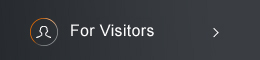Sponsored by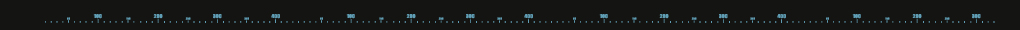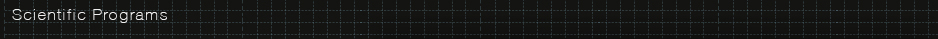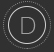Differential Equations and Stochastic Analysis

### Program Committee:

Jung-Chao Ban (NCCU), Chi-Hin Chan (NCTU), Long-Chi Chern (NCCU), Chun-Hsiung Hsia (NTU, committee chair),
Chun-Yen Shen (NTU), Kun-Chien Wu (NCKU).

Description of program:

The study of differential equations is one of the most traditional fields of mathematics in Taiwan. The NCTS Topical Program Differential Equations and Stochastic Analysis also aims to incorporate interdisciplinary research as one of its priorities, and its four main directions are: partial differential equations, dynamical systems, stochastic analysis and harmonic analysis. Partial differential equations are one of the most widely adopted mathematical tools in modern sciences. Members of this NCTS Topical Program group are interested in both the developments of the mathematical theory and scientific applications. This includes, for example, synchronization problem, kinetic theory, elliptic partial differential equations, fluid dynamics, inverse problem and reaction-diffusion equations. Interests of the research groups in dynamical systems include bifurcation theory and chaotic systems, ergodic theory, fractal geometry, arithmetic dynamical systems, and complex dynamical systems. The research interests of Harmonic analysis group include two weights problems for singular integrals, additive combinatorics and their connections to geometric measure theory. Probability theory also plays an important role in many other areas of mathematics, such as partial differential equations, analysis, and combinatorics. It provides the theoretical basis for statistics. In stochastic analysis at the NCTS, focus is placed on statistical mechanics, mathematical biology, finance, theoretical computer science, branching processes and SPDE.

Affiliated members:

Differential equations:

Chi-Hin Chan (NCTU), Chiun-Chuan Chen (NTU), I-Kun Chen (NTU), Zhi-You Chen (NCUE),
Ching-Hsiao Cheng (NCU), Jann-Long Chern (NTNU), Jong-Shenq Guo (TKU), Chun-Hsiung Hsia (NTU),
Hsin-Yuan Huang (NCTU), Hung-Wen Kuo (NCKU), Dong-Ho Tsai (NTHU), Jenn-Nan Wang (NTU),
Kun-Chien Wu (NCKU)

Dynamical systems:

Jung-Chao Ban (NCCU), Chih-Hung Chang (NUK), Kuo-Chang Chen (NTHU), Cheng-Hsiung Hsu (NCU)

Probability:

Guan-Yu Chen (NCTU), Long-Chi Chen (NCCU), Hao-Wei Huang (NSYSU), Chii-Ruey Hwang (AS),
Gi-Ren Liu (NCKU), Yuan-Chung Sheu (NCTU)

Analysis:

Chin-Cheng Lin (NCU), Chun-Yen Shen (NTU), Daniel Spector (OIST)Events2022/01/04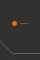On the Intersection of Dynamical Covering Sets with FractalsAbstract: Let $(X, \mathcal{B}, \mu, T, d)$ be a measure-preserving dynamical system with exp...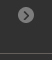2021/12/21Commutativity of Entropy of Noautonomous Markov Dystems on TreesAbstract: In this talk, the non-autonomous Markov systems (NMS) defined in trees are introduc...2021/12/07Badly Approximable and Non-recurrent Sets for Expanding Markov MIn this talk, we consider the asymptotic behaviors of the orbits of an expanding Markov system ([0, 1], f ) and prove that the badly approximable set is of fuAbstract: In this talk, we consider the asymptotic behaviors of the orbits of an expanding Ma...2021/11/23Large Deviation Principle of Multidimensional Multiple Averages on $\ mathbb{N}^d$Abstract: This paper establishes the large deviation principle (LDP) for multiple averages on...2021/11/09Li-Yorke Chaotic Sets for Continued Fractions and Beta TransformationsAbstract: We prove that there exists a scrambled set for the Gauss map with full Hausdorff di...2021/11/03Ground States for a Linearly Coupled Indefinite Schrodinger System with Steep Potential WellAbstract: In this talk, we are concerned with the investigation of a class of linearly couple...2021/11/02The Periodic Solutions of Scalar Delay Equations with Monotone Feedback and Even-odd SymmetryAbstract: Scalar delay differential equations are used to model phenomena in which a previous...2022/07/18Description: The purpose of the workshop is to introduce graduate students to fundamental res...Visitors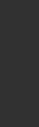Horng-Tzer Yau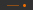Harvard Universityhtyau[AT]math.harvard.eduDecember 19, 2021 - January 5, 2022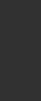Ju-Yi YenUniversity of Cincinnatiju-yi.yen[AT]uc.eduAugust 1 - December 31, 2021(C) 2021 National Center for Theoretical Sciences